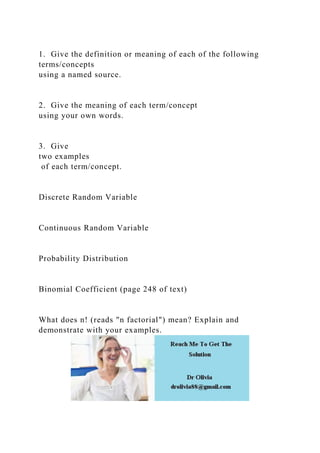Se está descargando tu SlideShare. ×

# 1.  Give the definition or meaning of each of the following termsco.docx

Anuncio
Anuncio
Anuncio
Anuncio
Anuncio
Anuncio
Anuncio
Anuncio
Anuncio
Anuncio
AnuncioCargando en…3
×

1 de 1 Anuncio

# 1.  Give the definition or meaning of each of the following termsco.docx

1.  Give the definition or meaning of each of the following terms/concepts
using a named source.

2.  Give the meaning of each term/concept

3.  Give
two examples
of each term/concept.

Discrete Random Variable

Continuous Random Variable

Probability Distribution

Binomial Coefficient (page 248 of text)

What does n! (reads "n factorial") mean? Explain and demonstrate with your examples.
.

1.  Give the definition or meaning of each of the following terms/concepts
using a named source.

2.  Give the meaning of each term/concept

3.  Give
two examples
of each term/concept.

Discrete Random Variable

Continuous Random Variable

Probability Distribution

Binomial Coefficient (page 248 of text)

What does n! (reads "n factorial") mean? Explain and demonstrate with your examples.
.

Anuncio
Anuncio

### 1.  Give the definition or meaning of each of the following termsco.docx

1. 1. 1. Give the definition or meaning of each of the following terms/concepts using a named source. 2. Give the meaning of each term/concept using your own words. 3. Give two examples of each term/concept. Discrete Random Variable Continuous Random Variable Probability Distribution Binomial Coefficient (page 248 of text) What does n! (reads "n factorial") mean? Explain and demonstrate with your examples.